## harmonic ratios and color

Tone and color frequencies and wavelengths have harmonic ratios {harmonic ratios, color}.

harmonics

Harmonic ratios have small integers in numerator and denominator. In increasing order of denominator, harmonic ratios are 1:1, 2:1, 3:2, 4:3, 5:3, 5:4, and so on.

color wavelengths

The purest red color is at light wavelength 683 nm, with orange at 608 nm, yellow at 583 nm, green at 543 nm, cyan at 500 nm, blue at 463 nm, and violet at 408 nm. Magenta can be at 380 nm or 760 nm.

color wavelength ratios

Color wavelength ratio for red/yellow, 683/583 = 1.17, and green/blue, 543/463 = 1.17, is 7/6 = 1.17 or 6/5 = 1.20. Color wavelength ratio for red/green, 683/543 = 1.26, and yellow/blue, 583/463 = 1.26, is 5/4 = 1.25. Color wavelength ratio for red/blue, 683/463 = 1.48, is 3/2 = 1.5. Color wavelength ratio for yellow/green, 583/543 = 1.07, is 13/12 = 1.085. Color wavelength ratio for red/violet, 683/408 = 1.67, and magenta/indigo, 725/435 = 1.67, is 5/3 = 1.67. See Figure 1.

color frequency ratios

Color frequency ratio for yellow/red, 518/436 = 1.19, and blue/green, 652/556 = 1.17, is 7/6 or 6/5. Color frequency ratio for green/red, 556/436 = 1.28, and blue/yellow, 652/518 = 1.26, is 5/4. Color frequency ratio for blue/red, 652/436 = 1.50, is 3/2. Color frequency ratio for green/yellow, 556/518 = 1.07, is 13/12. Color frequency ratio for violet/red, 740/436 = 1.70, and indigo/magenta, 694/420 = 1.66, is 5/3.

additive complementary colors

Additive complementary color pairs have same wavelength ratio, 4/3 = 1.33. Red/cyan is 683/500 = 1.37 to 650/500 = 1.30. Yellow/blue is 583/463 = 1.26 to 583/435 = 1.34. Chartreuse/indigo is 560/435 = 1.29 to 560/408 = 1.37. Magenta/green is 722/543 = 1.33.

Additive complementary-color triples have three color-pairs, whose average wavelength ratio is also 4/3. For three additive complementary colors, ratios are red/blue, 683/463 = 1.48, red/green, 683/543 = 1.26, and green/blue, 543/463 = 1.17. Arithmetic average is (1.5 + 1.25 + 1.2)/3 = 1.32. Geometric average is (1.5 * 1.25 * 1.2)^0.333 = 1.32. For three subtractive complementary colors, ratios are magenta/cyan, 722/500 = 1.45, magenta/yellow, 722/583 = 1.24, and yellow/cyan, 583/500 = 1.17. Average wavelength ratio is 4/3.

Three complementary colors have same relative values: red = 1.5, green = 1.2, and blue = 1, or magenta = 1.5, yellow = 1.2, and cyan = 1.

subtractive complementary colors

Because mixing darkens and blues colors, subtractive complementary color pairs have increasing wavelength ratios. Red/green is 683/543 = 1.26. Orange/blue is 608/463 = 1.31. Yellow/indigo is 583/435 = 1.34. Chartreuse/violet is 560/408 = 1.37.

color wavelength ratios starting at red

Starting with red at 1/1 = 683/683, orange is 8/7 = ~683/608, yellow is 7/6 = ~683/583, green is 5/4 = ~683/543, cyan is 4/3 = ~683/500, blue is 3/2 = ~683/463, violet is 5/3 = ~683/408, and magenta is 7/4 = ~683/380.

color wavelength ratios starting at green

Magenta is 2/3 = ~380/543. Violet is 3/4 = ~408/543. Blue is 5/6 = ~463/543. Cyan is 8/9 = ~500/543. Green is 1/1 = 543/543. Yellow is 17/16 = ~583/543. Orange is 9/8 = ~608/543. Red is 5/4 = ~683/543. Magenta is 4/3 = 720/543.

color wavelength ratios starting at red

Starting with red at 1/1 = 683/683, orange is 8/7 = ~683/608, yellow is 7/6 = ~683/583, green is 5/4 = ~683/543, cyan is 4/3 = ~683/500, blue is 3/2 = ~683/463, violet is 5/3 = ~683/408, and magenta is 7/4 = ~683/380.

color wavelength ratios starting and ending at magenta

On color circles, complementary colors are opposites. Complementary-color pairs have same wavelength ratio, so cyan/red = blue/yellow = magenta/green. Colors separated by same angle have same wavelength ratio, so yellow/red = green/yellow = cyan/green = blue/cyan = magenta/blue = red/magenta. Example color circle has red = 32, yellow = 16, green = 8, cyan = 4, blue = 2, and magenta = 1 and 64. Put into octave as exponentials, red = 2^0.83, yellow = 2^0.67, green = 2^0.5, cyan = 2^0.33, blue = 2^0.17, and magenta = 2^0 and 2^1. Put into octave, magenta = 2/1, red = 9/5, yellow = 8/5, green = 7/5, cyan = 5/4, blue = 9/8, and magenta = 1/1. Complementary colors have ratio 1.412 = ~7/5. Neighboring colors have ratio 1.125 = 9/8. Example wavelengths with these ratios are magenta = 750 nm, red = 668 nm, yellow = 595 nm, green = 531 nm, cyan = 473 nm, blue = 421 nm, and magenta = 375 nm, close to actual color wavelengths.

color harmonic ratios

Color frequency categories are at harmonic ratios: 48 Hz for red, 60 Hz for green, and 72 Hz for blue. 60/48 = 1.25 = 5/4. 72/48 = 1.5 = 3/2. 72/60 = 1.2 = 6/5. See Figure 2. Color-pair wavelength ratios have harmonic relations. Red/magenta = 7/4. Red/violet and magenta/indigo = 5/3. Red/blue = 3/2. Complementary colors red/cyan, yellow/blue, chartreuse/indigo, and magenta/green = 4/3. Red/green and yellow/blue = 5/4. Red/yellow and green/blue = 6/5 or 7/6. Red/orange = 8/7. Green/cyan = 9/8. Yellow/green = 13/12. See Figure 3.

Red, green, and blue add to make white. Magenta, cyan, and yellow add to make black. For red, green, and blue, and for magenta, cyan, and yellow, average of the three color-pair wavelength ratios is 4/3.

Looking at only primary colors red, green, and blue, color-pair wavelength ratios are red/blue 3/2, red/green 6/5, and green/blue 5/4. Red:green:blue relations have 6:5:4 ratios.

Looking at wavelength differences rather than wavelength ratios, magenta, red, orange, yellow, green, cyan, blue, and violet have approximately equal wavelength differences between adjacent colors. See Figure 2. Setting wavelength difference equal to one, color wavelengths form series 8, 7, 6, 5, 4, 3, 2, and 1. See Figure 3.

Assuming colors are like tones, colors can fit into one octave. Primary colors red, green, and blue, and complementary colors cyan, magenta, and yellow, respectively, are equally spaced in octave from 2^0 to 2^1. Magenta, red, yellow, green, cyan, blue, and magenta form series 6, 5, 4, 3, 2, 1, and 0. Magenta = 2^1, red = 2^0.83, yellow = 2^0.67, green = 2^0.5, cyan = 2^0.33, blue = 2^0.17, and magenta = 2^0. Adjacent colors have ratio 2^0.17 = 1.125 = 9/8. All complementary colors have the same ratio, 2^0.5. All complementary-color triples, such as red/green/blue, average 2^0.5. White, gray, and black have average color-pair wavelength ratio 2^0.5. In this arrangement, color-pair ratios are red/magenta ~ 9/5, yellow/magenta ~ 8/5, green/magenta ~ 7/5, cyan/magenta ~ 5/4, and blue/magenta ~ 9/8. See Figure 3. In this arrangement, whites, grays, and blacks are farthest from being octaves and so have dissonance. Other colors have smaller integer ratios and so more consonance. Color categories are at harmonic ratios.

multiple harmonics

One pair has two or three categories, like tone intervals or red/green or red/green/blue. Two pairs make six or seven categories, like octave whole tones or main spectrum colors. Three pairs make 12 categories, like octave half tones or major spectrum colors. Four pairs make 24 categories, like octave quarter tones or major and minor spectrum colors.

summary

Using physical-color wavelengths, wavelength ratios are red/magenta = 7/4, red/violet = magenta/indigo = 5/3, red/blue = 3/2, red/cyan = yellow/blue= chartreuse/indigo = magenta/green = 4/3, red/green = yellow/blue = 5/4, red/yellow = green/blue = 6/5 or 7/6, red/orange = 8/7, green/cyan = 9/8, and yellow/green = 13/12.

Additive complementary-color pairs, such as red/cyan, yellow/blue, chartreuse/indigo, and magenta/green, have same 4/3 wavelength-ratio.

For red, green, and blue additive complementary colors, average of the three wavelength ratios, red/blue, red/green, and green/blue, is 4/3. For magenta, cyan, and yellow subtractive complementary colors, average of the three wavelength ratios, magenta/cyan, magenta/yellow, and yellow/cyan, is 4/3.

These intervals are harmonic musical tones in an octave: C, E, and G in the key of C. Blue and red make a major fifth interval. Blue and green make a minor third interval. Green and red make a major third interval.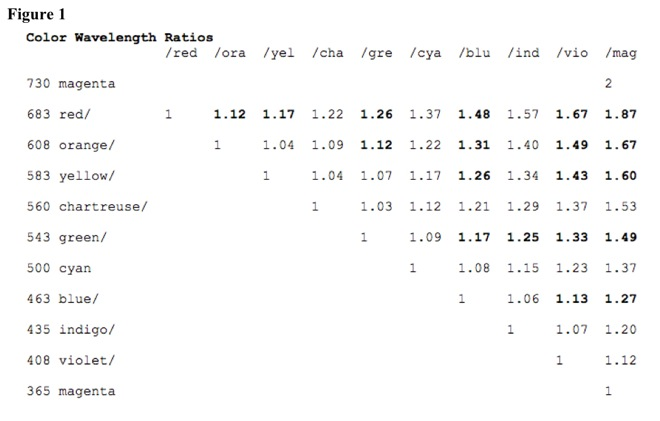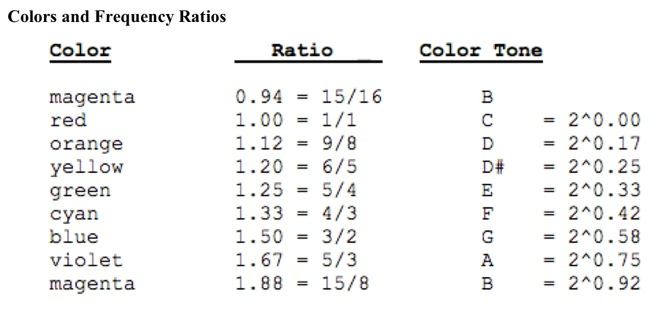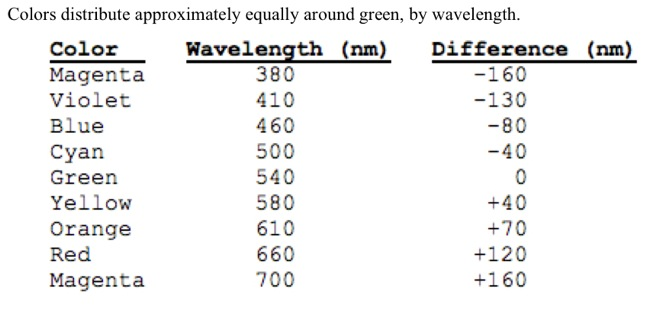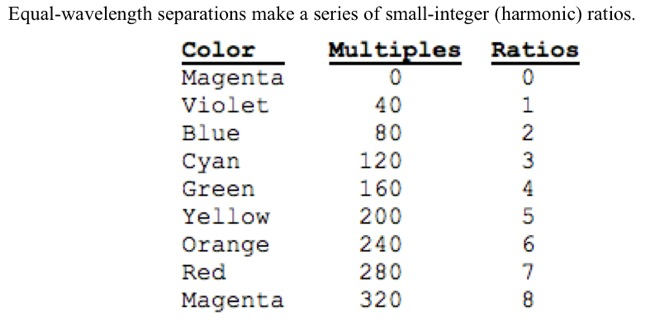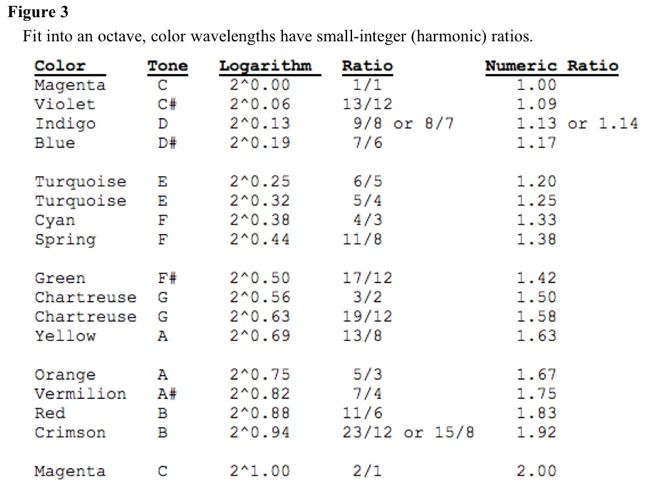#### Related Topics in Table of Contents

Consciousness>Consciousness>Speculations>Sensation>Mathematics>Color

#### Whole Section in One File

1-Consciousness-Speculations-Sensation-Mathematics-Color

Drawings

#### Contents and Indexes of Topics, Names, and Works

Outline of Knowledge Database Home Page

Contents

Glossary

Topic Index

Name Index

Works Index

Search Form

#### Database Information, Disclaimer, Privacy Statement, and Rights

Description of Outline of Knowledge Database

Notation

Disclaimer

Copyright Not Claimed

Privacy Statement

#### References and Bibliography

Consciousness Bibliography

#### Technical Information

Date Modified: 2015.0626

Description: harmonic ratios and color - Tone and color frequencies and wavelengths have harmonic ratios {harmonic ratios, color}. **#**harmonics *****Harmonic ratios have small integers in numerator and denomin